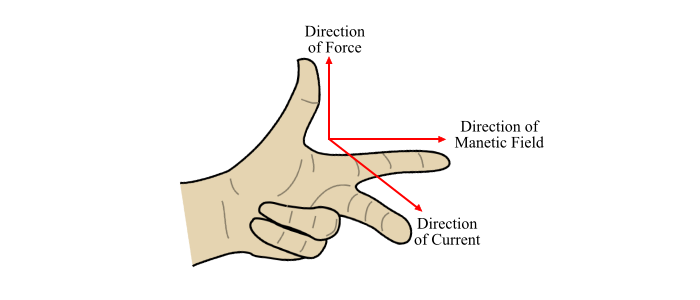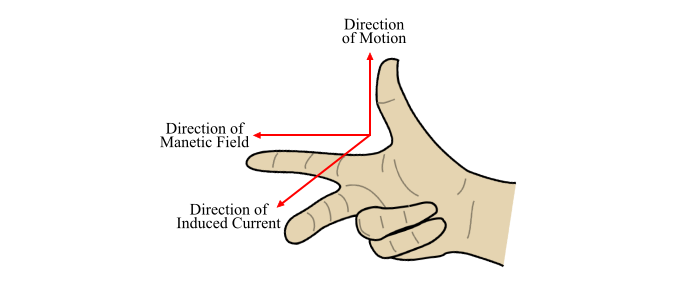# Fleming’s Left-Hand and Right-Hand Rules Explained

An English electrical engineer and physicist Sir John Ambrose Fleming established two rules, known as Fleming's Left-Hand Rule and Fleming's Right-Hand Rule which are widely used in electrical machines.

When a current carrying conductor is placed in a magnetic field, a mechanical force acts on the conductor. The direction this force is determined by Fleming's Left-Hand Rule. The left-hand rule is mainly applicable in electric motors.

Also, when a conductor is moved in a magnetic field, an emf is induced in the conductor due to which an electric current flows in the conductor. The direction this induced current is determined by Fleming's Right-Hand Rule. The right-hand rule is applicable in electric generators.

## Fleming's Left-Hand Rule

Fleming's Left-Hand Rule is stated as follows −

"Stretch out the forefinger, middle finger and thumb of your left-hand so that they are at right angle to one another. If the forefinger points in the direction of magnetic field, the middle finger point in the direction of current, then the thumb will point in the direction of the force".Fleming's left-hand rule is particularly suitable to find the direction of force on a current conductor when it is placed in a magnetic field (as in an electric motor).

## Fleming's Right-Hand Rule

Fleming's Right-Hand Rule is stated as follows −

"Stretch out the forefinger, middle finger and thumb of your right-hand so that they are at right angle from one another. If the forefinger points in the direction of magnetic field, the thumb point in the direction of motion of the conductor, then the middle finger will point in the direction of induced emf (or current)".Fleming's Right-Hand Rule is suitable to find the direction of induced emf and hence the current when conductor moves at right angle to a stationary magnetic field (as in an electric generator).